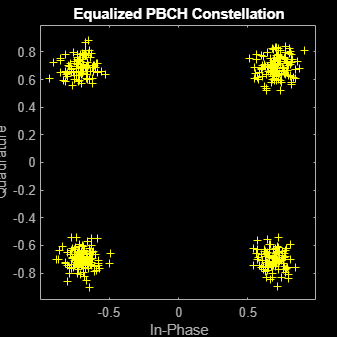# nrEqualizeMMSE

Minimum mean-squared error (MMSE) equalization

## Syntax

``````[eqSym,csi] = nrEqualizeMMSE(rxSym,hest,nVar)``````

## Description

example

``````[eqSym,csi] = nrEqualizeMMSE(rxSym,hest,nVar)``` applies MMSE equalization to the extracted resource elements of a physical channel `rxSym` and returns the equalized symbols in `eqSym`. The equalization process uses the estimated channel information `hest` and the estimate of the received noise variance `nVar`. The function also returns the soft channel state information `csi`.```

## Examples

collapse all

Perform MMSE equalization on extracted resource elements of the physical broadcast channel (PBCH).

Create symbols and indices for a PBCH transmission.

```ncellid = 146; v = 0; E = 864; cw = randi([0 1],E,1); pbchTxSym = nrPBCH(cw,ncellid,v); pbchInd = nrPBCHIndices(ncellid);```

Generate an empty resource array for one transmitting antenna. Populate the array with the PBCH symbols by using the generated PBCH indices.

```carrier = nrCarrierConfig('NSizeGrid',20); P = 1; txGrid = nrResourceGrid(carrier,P); txGrid(pbchInd) = pbchTxSym;```

Perform OFDM modulation.

`txWaveform = nrOFDMModulate(carrier,txGrid);`

Create channel matrix and apply channel to transmitted waveform.

```R = 4; H = dftmtx(max([P R])); H = H(1:P,1:R); H = H / norm(H); rxWaveform = txWaveform * H;```

Create channel estimate.

```hEstGrid = repmat(permute(H.',[3 4 1 2]),[240 4]); nEst = 0.1;```

Perform OFDM demodulation.

`rxGrid = nrOFDMDemodulate(carrier,rxWaveform);`

To prepare for PBCH decoding, use `nrExtractResources` to extract symbols from received and channel estimate grids. Plot the received PBCH constellation.

```[pbchRxSym,pbchHestSym] = nrExtractResources(pbchInd,rxGrid,hEstGrid); figure; plot(pbchRxSym,'o:'); title('Received PBCH Constellation');```Decode the PBCH with the extracted resource elements. Plot the equalized PBCH constellation.

```[pbchEqSym,csi] = nrEqualizeMMSE(pbchRxSym,pbchHestSym,nEst); pbchBits = nrPBCHDecode(pbchEqSym,ncellid,v); figure; plot(pbchEqSym,'o:'); title('Equalized PBCH Constellation');```## Input Arguments

collapse all

Extracted resource elements of a physical channel, specified as an NRE-by-R numeric matrix. NRE is the number of resource elements extracted from each K-by-L plane of the received grid. K is the number of subcarriers and L is the number of OFDM symbols. R is the number of receive antennas.

Data Types: `double`
Complex Number Support: Yes

Estimated channel information, specified as an NRE-by-R-by-P numeric array. NRE is the number of resource elements extracted from each K-by-L plane of the received grid. K is the number of subcarriers and L is the number of OFDM symbols. R is the number of receive antennas. P is the number of transmission planes.

Data Types: `double`
Complex Number Support: Yes

Estimated noise variance, specified as a real nonnegative scalar.

Data Types: `double`

## Output Arguments

collapse all

Equalized symbols, returned as an NRE-by-P numeric matrix. NRE is the number of resource elements extracted from each K-by-L plane of the received grid. K is the number of subcarriers and L is the number of OFDM symbols. P is the number of transmission planes.

Data Types: `double`
Complex Number Support: Yes

Soft channel state information, returned as an NRE-by-P numeric matrix. NRE is the number of resource elements extracted from each K-by-L plane of the received grid. K is the number of subcarriers and L is the number of OFDM symbols. P is the number of transmission planes.

Data Types: `double`
Complex Number Support: Yes

## Version History

Introduced in R2018b